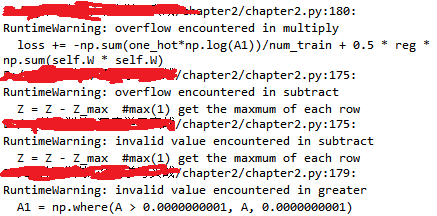``````     def loss(self,X,y,reg):
loss = 0.0
dW = np.zeros_like(self.W)

num_train = X.shape
num_class = self.W.shape

one_hot = np.zeros(shape = (num_train,num_class))
y = y.astype('int64')
one_hot[np.arange(0,num_train), y] = 1

Z = X.dot(self.W)
Z_max = np.max(Z,axis = 1,keepdims = True) #Z_max = Z.max(1).reshape(num_train,1)
Z = Z - Z_max  #max(1) get the maxmum of each row
score_E = np.exp(Z)
Sum = np.sum(score_E,axis = 1,keepdims = True)#score_E.sum(axis = 1).reshape(num_train,1)  # sum of each row
A = score_E/Sum   #score of every class of each training_example
A1 = np.where(A > 0.0000000001, A, 0.0000000001)
loss += -np.sum(one_hot*np.log(A1))/num_train + 0.5 * reg * np.sum(self.W * self.W)
dW += -np.dot(X.T, one_hot - A) / num_train + reg * self.W
return loss,dW
``````2个回答

softmax你算的不对，公式你看下：https://www.cnblogs.com/liuyu124/p/7332476.html

e的x次方，若x较大结果会溢出的，所以一般对所有的x值同时减去x_max，保证结果不溢出。python如何自定义权重损失函数？

one-hot独热编码用softmax分类器如何实现

one-hot独热编码用softmax分类器如何实现 ！！在函数调用中实现，还是输出直接编码成独热编码直接输出就可以啊，还有我的输入用的是矩阵形式，输出是编码形式，比如是1000000 0100000等，就不能作为矩阵输出，这样输入输出形式不一样可以吗？（输入是矩阵，输出是数组）

softmax分类器共有10类，训练数据中没有第1类，测试时怎样正确预测第1类的样本？

tensorflow训练网络时精度很高，在训练时查看softmax之后的概率值和实际的标签值却对应不上

python测试集结果调取问题

test_acc = sess.run(accr,feed_dict=feeds_test) 这个语句是用来调出测试准确率的，如何才能调出测试期间对每一个样本的预测数值？？？？ x=tf.placeholder("float", [None,784]) #placeholder 占位，不赋给x实际值,784 像素值， None无穷样本 y=tf.placeholder("float", [None,10]) #10个分类目标 W=tf.Variable(tf.zeros([784,10])) b=tf.Variable(tf.zeros()) #tf.zeros 初始化 actv= tf.nn.softmax(tf.matmul(x,W)+b) #cost function cost=tf.reduce_mean(-tf.reduce_sum(y*tf.log(actv), reduction_indices=1)) learning_rate=0.01 optm= tf.train.GradientDescentOptimizer(learning_rate).minimize(cost) print ('1') pred=tf.equal(tf.argmax(actv, 1), tf.argmax(y, 1)) #ACCURACY accr=tf.reduce_mean(tf.cast(pred,"float")) #INITIALIZER init=tf.global_variables_initializer() training_epochs = 50 #所有样本迭代次数=50 batch_size = 100 #每次迭代用多少样本 display_step = 5 #展示 sess=tf.Session() sess.run(init) #跑初始化 for epoch in range (training_epochs): avg_cost=0 num_batch=int(mnist.train.num_examples/batch_size) for i in range (num_batch): batch_xs, batch_ys= mnist.train.next_batch(batch_size) #一步一步的往下找 sess.run(optm, feed_dict={x: batch_xs, y: batch_ys}) feeds={x:batch_xs, y: batch_ys} avg_cost += sess.run (cost, feed_dict=feeds)/num_batch #display if epoch % display_step == 0: feeds_train = {x: batch_xs, y: batch_ys} feeds_test = {x: mnist.test.images, y: mnist.test.labels} train_acc = sess.run(accr, feed_dict=feeds_train) #feed_dict 针对place holder占位 test_acc = sess.run(accr,feed_dict=feeds_test) print ("Epoch: %03d/%03d cost: %.9f train_acc: %.3f test_acc: %.3f" % (epoch, training_epochs, avg_cost, train_acc, test_acc))

softmax分类器，分类概率都是nan是怎么回事？

Python Tensorflow中dense问题

tf.layers.dense中units的参数设定依据什么规则？是维数越大越精确吗？刚刚开始学，希望能细讲下谢谢

toolbox 搭建的SAE 模型中，怎么加入softmax分类器作为输出,是直接把nn.output选为softmax就可以了吗，用MATLAB来搭建SAE是分第一训练层，第二训练层，softmax训练层，在toolbox中不需要是吗？

1. 因为我只需要CNN的这个特征向量，但是我找不到从哪里打断点获取，请问哪位大神知道如何在MATLAB中获取这个CNN的特征向量呢？多谢~

pytoch交叉熵函数 IndexError: Dimension out of range (expected to be in range of [-1, 0], but got 1)

python keras sequential输入

Java基础知识面试题（2020最新版）

Linux 会成为主流桌面操作系统吗？

loonggg读完需要3分钟速读仅需 1 分钟大家好，我是你们的校长。我之前讲过，这年头，只要肯动脑，肯行动，程序员凭借自己的技术，赚钱的方式还是有很多种的。仅仅靠在公司出卖自己的劳动时...

MySQL数据库面试题（2020最新版）

Redis核心原理与应用实践

Redis核心原理与应用实践 在很多场景下都会使用Redis，但是到了深层次的时候就了解的不是那么深刻，以至于在面试的时候经常会遇到卡壳的现象，学习知识要做到系统和深入，不要把Redis想象的过于复杂，和Mysql一样，是个读取数据的软件。 有一个理解是Redis是key value缓存服务器，更多的优点在于对value的操作更加丰富。 安装 yum install redis #yum安装 b...

!大部分程序员只会写3年代码

2020阿里全球数学大赛：3万名高手、4道题、2天2夜未交卷

HTTP与HTTPS的区别